A tree is a type of graph data structure composed of nodes and edges. Its main properties are

• It is acyclic (doesn't contain any cycles);
• There exists a path from the root to any node;
• Has `N - 1` edges, where `N` is the number of nodes in the tree; and
• Each node has exactly one parent node with the exception of the root node

Here are some examples of trees:

We have used terms above that you may be unfamiliar with. Here are there definitions and an example to accompany them:

Here's the summary:

• Internal node: every node in a tree that has at least one child node.
• Leaf node: every node in a tree that has no child nodes.
• Ancestor: all the nodes that are between the path from the root to the current node are the ancestors of the current node. An ancestor node of the current node is either the parent of the current node or the parent of another ancestor of the node.
• Descendent: all the nodes that are reachable from the current node when moving down the tree are the descendants of the current node. A descendant of the current node is either a child of the node or a child of another descendant of the node.
• Level: the number of ancestors from the node to the root nodes.

## Binary Tree

An n-ary tree is a tree in which each node has no more than `n` children. A binary tree is a type of n-nary tree with `n = 2`, so every node in a binary tree has 0 to 2 children.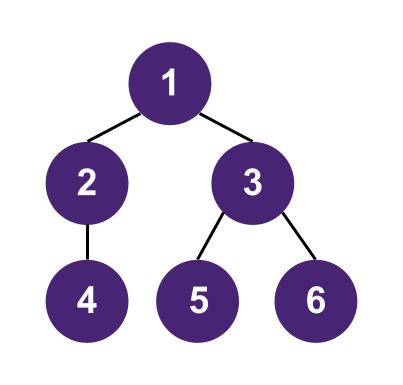### Binary Tree implementation in Python, Java, C++ and Javascript

``````1class Node:
2  def __init__(self, val):
3    self.val = val
4    self.left = None
5    self.right = None
6``````
``````1class Node<T> {
2  public T val;
3  public Node<T> left;
4  public Node<T> right;
5
6  public Node(T val) {
7      this(val, null, null);
8  }
9
10  public Node(T val, Node<T> left, Node<T> right) {
11      this.val = val;
12      this.left = left;
13      this.right = right;
14  }
15}
16``````
``````1template <typename T>
2struct Node {
3  T val;
4  Node<T>* left;
5  Node<T>* right;
6
7  explicit Node(T val, Node<T>* left = nullptr, Node<T>* right = nullptr)
8      : val{val}, left{left}, right{right} {}
9
10  ~Node() {
11      delete left;
12      delete right;
13  }
14};
15``````
``````1class Node {
2  constructor(val, left = null, right = null) {
3    this.val = val;
4    this.left = left;
5    this.right = right;
6  }
7}
8``````
``````1class Node<T>
2{
3    public T val;
4    public Node<T> left;
5    public Node<T> right;
6
7    public Node(T val)
8    {
9        this.val = val;
10    }
11
12    public Node(T val, Node<T> left, Node<T> right)
13    {
14        this.val = val;
15        this.left = left;
16        this.right = right;
17    }
18}
19``````

### Full, Complete and Perfect Binary Trees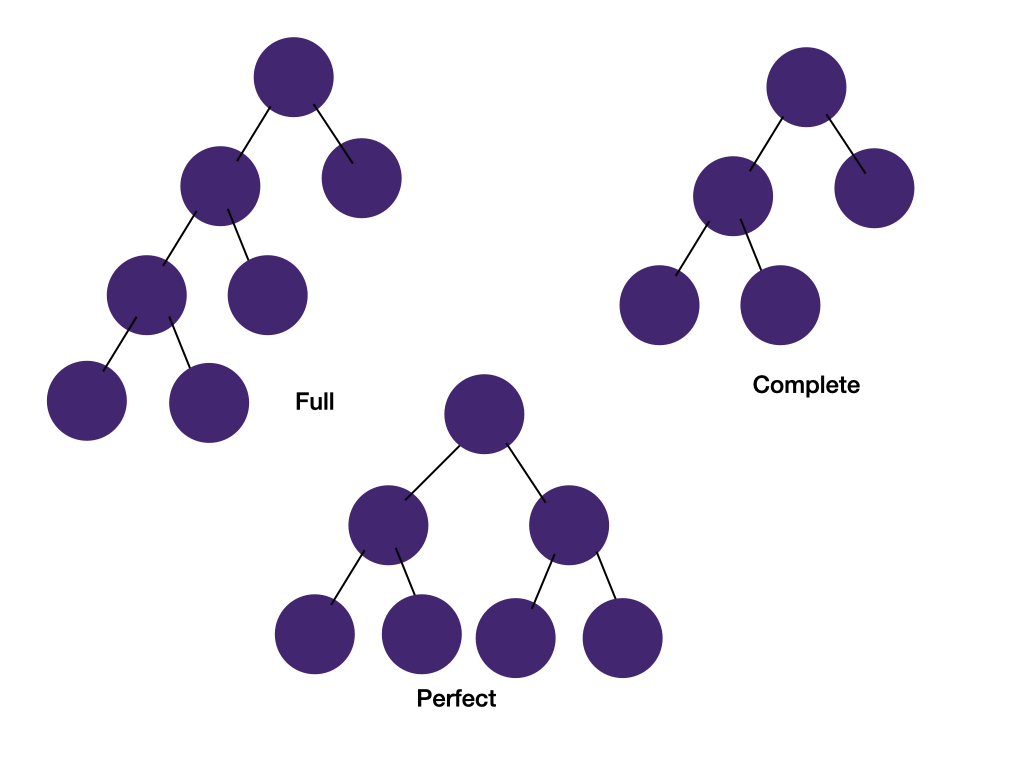#### Full binary tree

Every node has 0 or 2 children.

#### Complete binary tree

All levels are completely filled except possibly the last level and all nodes in the last level are as far left as possible. This may sound like an odd concept. We will see its usage in the heap section.

#### Perfect binary tree

All internals nodes have two children and all leaf nodes have the same level. Perfect binary trees are often used to estimate time complexity for combinatorial problems where the search space is a perfect binary tree.

A perfect binary tree has the following properties:

• Number of nodes in a perfect binary tree is `2^n-1` where `n` is the number of levels. Calculation: The first level has 1 node, the root. The next level has 2 nodes. The following levels have 4, 8, 16.. nodes. This is a geometric sequence and the sum is `a(1-r^n)/(1-r)`. Plug in `a = 1` and `r = 2` and we get `2^n-1`.
• Number of internal nodes = number of leaf nodes - 1. Calculation: A perfect binary tree of height `n+1` will have `2^n` leaf nodes. The internal nodes in the tree of height `n+1` form a perfect binary tree of height `n` with `2^n-1` total nodes. Comparing the two values, we see that # of internal nodes = # leaf nodes - 1.
• Total number of nodes = 2 * number of leaf nodes - 1. This is a derivative of property #2 and the fact that the total number of nodes = number of leaf nodes + number of internal nodes. So the number of total nodes and leaf nodes are both `O(2^n)`

### Binary Search Tree

A binary search tree (BST) is a special type of binary tree, in which every nodes follows the ordering property of `all left descendents < node < all right descendents`. Learn more about BST in Binary Search Tree Intro.

### Balanced Binary Tree

Every node in a balanced binary tree fullfills the condition--the height difference of the left and right subtree of the node is not more than 1. Searching, insertion, and deletion in a balanced binary tree takes `O(log n)` instead of `O(n)` in an unbalanced binary tree. This is an example of a balanced binary tree: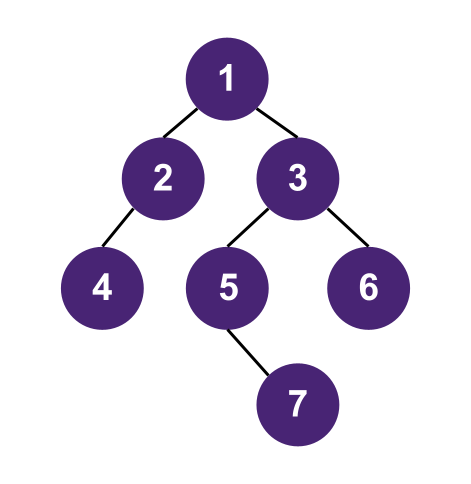Some common types of balanced binary trees are red-black trees and AVL trees. It's good to be aware of these trees but they are too complex to be asked in a coding interview.

## Tree Traversal

### In-order Traversal

In-order traversal visits the left branch first, then the current node, and finally the right branch. The diagram below shows the traversal order of an in-order traversal on a binary tree.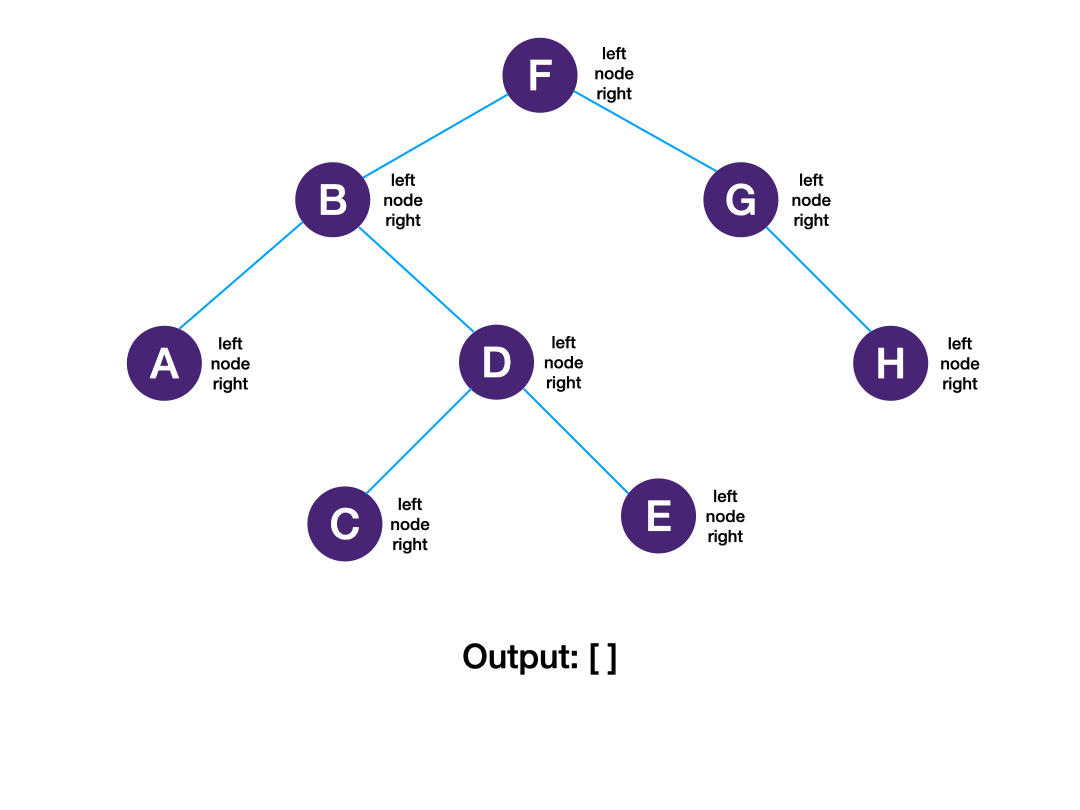Below is a demonstration of how you would perform in-order traversal on a binary tree using recursion

### Pre-order Traversal

Pre-order traversal visits the current node first, then the left subtree, and finally the right subtree. The diagram below shows the traversal order of a pre-order traversal on a binary tree.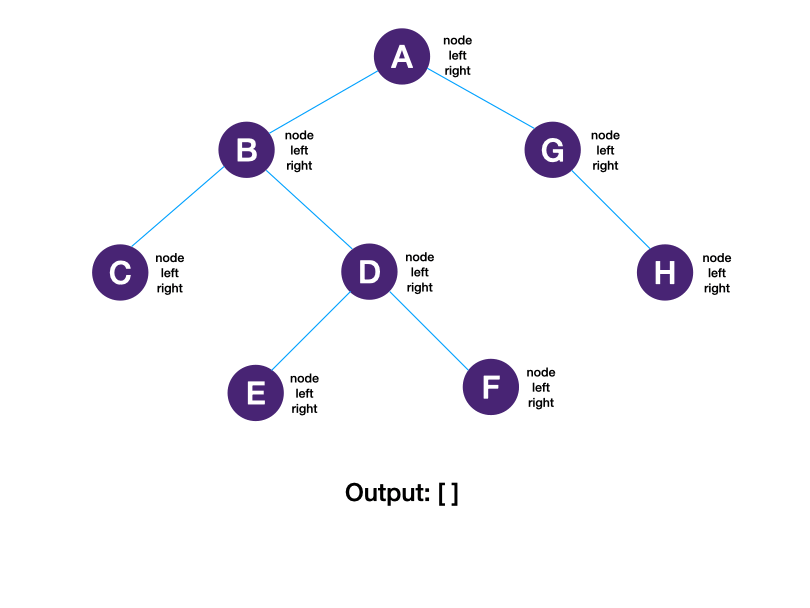### Post-order Traversal

Post-order traversal visits the left subtree first, then the right subtree, and finally the current node. The diagram below shows the traversal order of a post-order traversal on a binary tree.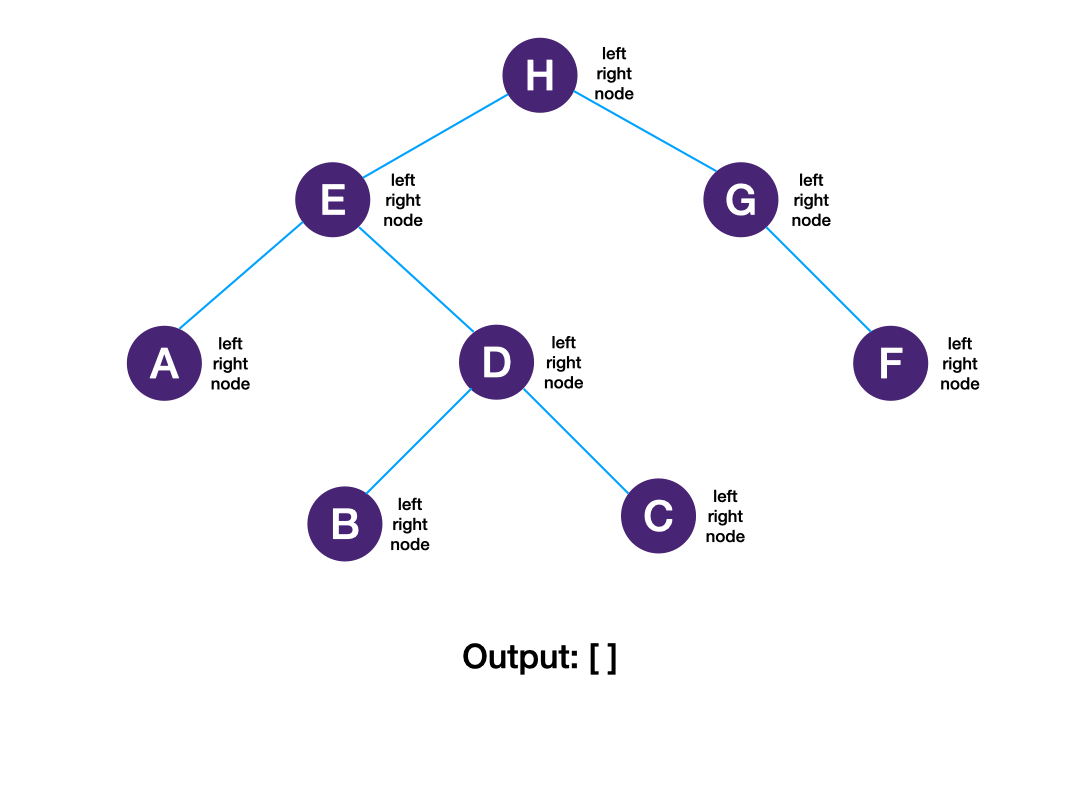## How AlgoMonster encode trees

When you work through our lessons, you will encounter many problems involving trees. It is helpful to know how we encode trees, so you may be able to make up your own custom tree input to test your code.

### How AlgoMonster encode binary trees

We represent a binary tree as a string. The values of each node are separated by an empty space, and null nodes are represented by `"x"`. The function `build_tree()` in our driver code, which processes a given string into a binary tree, fills the tree with pre-order traversal. This example demonstrates how we represent and encode a binary tree input: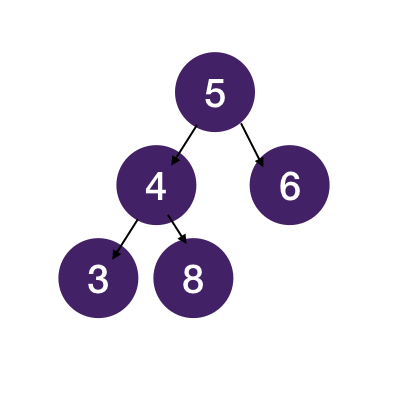The string representation of this tree is `5 4 3 x x 8 x x 6 x x`, and this is how `build_tree()` builds a binary tree from the string input:

### How AlgoMonster encode non-binary trees

For trees other than binary trees, we define the tree nodes as:

``````1class Node:
2    def __init__(self, val, children=None):
3        if children is None:
4            children = []
5        self.val = val
6        self.children = children``````

Similarly, we also represent a non-binary tree as a string. The values of each node and the number of its children are separated by an empty space. The function `build_tree()` in our driver code, which processes a given string into a tree, also fills the tree with pre-order traversal. This example demonstrates how we represent and encode a non-binary tree input: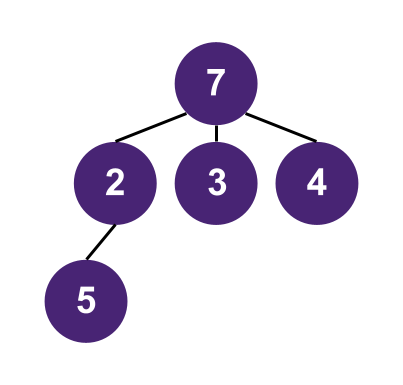The string representation of the tree shown above is `7 3 2 1 5 0 3 0 4 0`, and this is how `build_tree()` builds a tree from the string input:

Still not clear? Submit the part you don't understand to our editors.